ＳＱＬの窓

## 2022年01月02日

### 「送る」からファイルのダンプ

```GitHub※コマンドプロンプトの操作
Q : 終了します。
スペースキー : 次ページを表示します。
Enterキー : 次の行を表示します

dump.vbs
```
```if WScript.Arguments.Count = 1 then
strMessage = "送るから実行して下さい" & vbCrLf & vbCrLf
strMessage = strMessage & "※ リンク先の最後の数字はコマンドプロンプトの行数です　　　" & vbCrLf
strMessage = strMessage & "※ プロパティよりウインドウを最大化する方法もあります　　　" & vbCrLf
Call MsgBox(strMessage,0,"lightbox")
Wscript.Quit
end if

Set WshShell = CreateObject( "WScript.Shell" )
Set Fso = CreateObject( "Scripting.FileSystemObject" )

strCurPath = WScript.ScriptFullName
Set obj = Fso.GetFile( strCurPath )
Set obj = obj.ParentFolder
strCurPath = obj.Path

strCommand = "cmd /k mode CON lines="&WScript.Arguments(0)&" & cscript.exe """ & _
strCurPath & "\dump_c.vbs"" """ & WScript.Arguments(1) & """ | more & pause"
Call WshShell.Run( strCommand )

```
```
dump_c.vbs
```
```' ****************************************************
' ファイルを１６進数でダンプします
' ****************************************************
Dim Fs,Stream
Dim InFile
Dim Kana
Dim KjFlg
Kana = Array( _
"｡","｢","｣","､","･","ｦ","ｧ","ｨ","ｩ","ｪ","ｫ","ｬ","ｭ","ｮ","ｯ", _
"ｰ","ｱ","ｲ","ｳ","ｴ","ｵ","ｶ","ｷ","ｸ","ｹ","ｺ","ｻ","ｼ","ｽ","ｾ","ｿ", _
"ﾀ","ﾁ","ﾂ","ﾃ","ﾄ","ﾅ","ﾆ","ﾇ","ﾈ","ﾉ","ﾊ","ﾋ","ﾌ","ﾍ","ﾎ","ﾏ", _
"ﾐ","ﾑ","ﾒ","ﾓ","ﾔ","ﾕ","ﾖ","ﾗ","ﾘ","ﾙ","ﾚ","ﾛ","ﾜ","ﾝ","ﾞ","ﾟ" )

Set Fs = CreateObject( "Scripting.FileSystemObject" )
Set Stream = CreateObject("ADODB.Stream")

InFile = WScript.Arguments(0)

Dim LineBuffer,DispBuffer,CWork,nCnt,strBuff,i,j

if not Fs.FileExists( InFile ) then
Wscript.Echo "ファイルが存在しません"
Wscript.Quit
end if

' ------------------------------------------------------
' Stream のオープン
Stream.Open

' ------------------------------------------------------
' Stream タイプの指定
Stream.Type = 1		' StreamTypeEnum の adTypeBinary

' ------------------------------------------------------
' 既存ファイルの内容を Stream に読み込む

' ------------------------------------------------------
' バイナリ型の Stream オブジェクトからを読み取って加工
Bcnt = 0
nCnt = 0
KjFlg = ""

Do while not Stream.EOS

if ( nCnt MOD 16 ) = 0 then
Wscript.Echo "          0  1  2  3  4  5  6  7" _
& "  8  9  A  B  C  D  E  F"
Wscript.Echo "--------------------------------" _
& "------------------------------------------"
end if

' 16 バイトの読込

strBuff = ""
For i = 1 to LenB( LineBuffer )
CWork = MidB(LineBuffer,i,1)
Cwork = AscB(Cwork)
Cwork = Hex(Cwork)
Cwork = Ucase(Cwork)
Cwork = Right( "0" & Cwork, 2 )
DispBuffer = DispBuffer & Cwork & " "
strBuff = strBuff & CharConv( Cwork )
Next

Wscript.Echo _
Right( _
"00000000" & Ucase(Hex( nCnt * 16 )), 8 _
) & " " & _
Left(DispBuffer & String(49," "), 49 ) & strBuff
DispBuffer = ""

nCnt = nCnt + 1

Loop

' ------------------------------------------------------
' Stream を閉じる
Stream.Close

Set Stream = Nothing
Stream = Empty
Set Fs = Nothing
Fs = Empty

' ****************************************************
' 生データのテキスト
' ****************************************************
function CharConv( HexCode )

Dim nCode

nCode = Cint( "&H" & HexCode )

if KjFlg = "" then
if &H81 <= nCode and nCode <= &H84 or _
&H88 <= nCode and nCode <= &H9f or _
&HE0 <= nCode and nCode <= &HEA then
KjFlg = HexCode
CharConv = ""
Exit Function
end if
else
if HexCode <> "00" then
KjFlg = KjFlg & HexCode
CharConv = Chr( Cint( "&H" & KjFlg ) )
else
CharConv = ".."
end if
KjFlg = ""
Exit Function
end if

if 0 <= nCode and nCode <= &H1F then
CharConv = "."
end if
if &H20 <= nCode and nCode <= &H7E then
CharConv = Chr(nCode)
end if
if &H7F <= nCode and nCode <= &HA0 then
CharConv = "."
end if
if &HA1 <= nCode and nCode <= &HDF then
CharConv = Kana(nCode-&HA1)
end if
if &HE0 <= nCode and nCode <= &HFF then
CharConv = "."
end if

end function

```
```
エクスプローラで SendTo フォルダに移動するには、アドレスバーに sendto と直接入力します。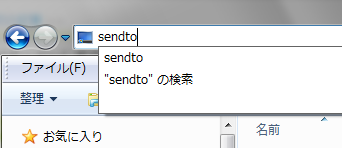```

## 2019年02月01日

### VBScript : ファイルのフルパスをクリップボードへ( ダブルクォートなし )

```エクスプローラで、SHIFT キーを押しながら右クリックすると『パスとしてコピー』がありますが、ダブルクォートが付加されています( たいていはそのほうがいいのですが )ので、ダブルクォートのないパスを取得します▼ こんな感じで取得されます
"C:\Program Files\7-Zip\7-zip.dll"

filepath.vbs( SendTo ディレクトリに置いてください )

```
```Set WshShell = Wscript.CreateObject("WScript.Shell")
Set Fso = Wscript.CreateObject("Scripting.FileSystemObject")

strTemp = WshShell.ExpandEnvironmentStrings("%temp%")
strPath = strTemp & "\__clipCommand.tmp"

Set objHandle = Fso.OpenTextFile( strPath, 2, True )
objHandle.Write Wscript.Arguments(0)

Call WshShell.Run( "cmd.exe /c clip < """ & strPath & """", 0, True )
```
```▼ こうなります
C:\Program Files\7-Zip\7-zip.dll

filename.vbs( SendTo ディレクトリに置いてください )

```
```Set WshShell = Wscript.CreateObject("WScript.Shell")
Set Fso = Wscript.CreateObject("Scripting.FileSystemObject")

strTemp = WshShell.ExpandEnvironmentStrings("%temp%")
strPath = strTemp & "\__clipCommand.tmp"

Set objHandle = Fso.OpenTextFile( strPath, 2, True )
strName = Wscript.Arguments(0)
aPath = Split(strName,"\")
strName = aPath(Ubound(aPath))
objHandle.Write strName

Call WshShell.Run( "cmd.exe /c clip < """ & strPath & """", 0, True )

```
```▼ こうなります
7-zip.dll

さらに以下では、複数ファイルを選択した場合のファイル名部分だけを取り出して複数行としてコピーします( 但しあまり大量のファイルは元々の文字列の制限によりエラーとなります

filelist.vbs( SendTo ディレクトリに置いてください )

```
```Set WshShell = Wscript.CreateObject("WScript.Shell")
Set Fso = Wscript.CreateObject("Scripting.FileSystemObject")

str = ""
For I = 0 to Wscript.Arguments.Count-1
aData = Split( Wscript.Arguments(I), "\" )
str = str & aData(Ubound(aData)) & vbCrLf
Next

strTemp = WshShell.ExpandEnvironmentStrings("%temp%")
strPath = strTemp & "\__clipCommand.tmp"

Set objHandle = Fso.OpenTextFile( strPath, 2, True )
objHandle.Write str
Call WshShell.Run( "cmd.exe /c clip < """ & strPath & """", 0, True )

```
```▼ ファイルが多すぎて起きるエラー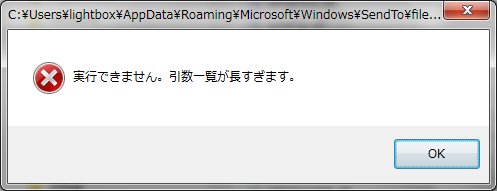▼ うまくいくとこんな感じです
nslookup.exe
ntdll.dll
odbc32.dll
ole32.dll

エクスプローラで SendTo フォルダに移動するには、アドレスバーに sendto と直接入力します。テンポラリフォルダは、%temp% と入力して下さい。コードを直接ダウンロードした場合は、右クリックのプロパティより『許可する』にチェックしておきます。filepath.vbs

Wscript.Arguments(0) が目的の文字列なので、この前後に任意の文字列を追加します。
```
```Set WshShell = Wscript.CreateObject("WScript.Shell")
Set Fso = Wscript.CreateObject("Scripting.FileSystemObject")

strTemp = WshShell.ExpandEnvironmentStrings("%temp%")
strPath = strTemp & "\__clipCommand.tmp"

Set objHandle = Fso.OpenTextFile( strPath, 2, True )
objHandle.Write "`" & Wscript.Arguments(0) & "`"

Call WshShell.Run( "cmd.exe /c clip < """ & strPath & """", 0, True )

```
```
filelist.vbs

```
```Set WshShell = Wscript.CreateObject("WScript.Shell")
Set Fso = Wscript.CreateObject("Scripting.FileSystemObject")

str = ""
For I = 0 to Wscript.Arguments.Count-1
aData = Split( Wscript.Arguments(I), "\" )
str = str & "これは" & aData(Ubound(aData)) & "です" & vbCrLf
Next

strTemp = WshShell.ExpandEnvironmentStrings("%temp%")
strPath = strTemp & "\__clipCommand.tmp"

Set objHandle = Fso.OpenTextFile( strPath, 2, True )
objHandle.Write str
Call WshShell.Run( "cmd.exe /c clip < """ & strPath & """", 0, True )

```
```

```

## 2015年03月06日

### 送る( SendTo )フォルダ 用 VBScript 基本ソースコード

```
sendto_test.vbs
```
```str = ""
For I = 0 to Wscript.Arguments.Count-1
str = str & Wscript.Arguments(I) & vbCrLf
Next
Wscript.Echo str

```
```『送る( SendTo )に』置いて処理すると、選択されたファイルのフルパスをメッセージボックスに表示します。但し、文字列量には限界があります。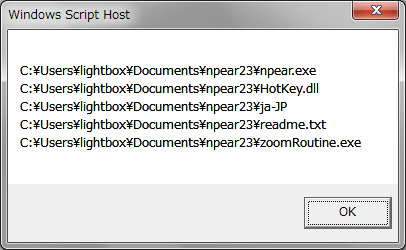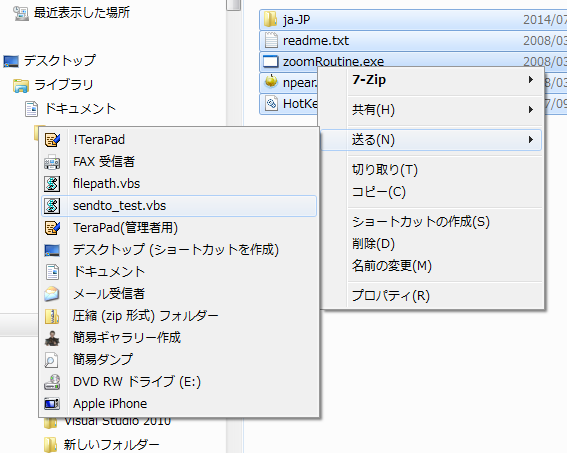エクスプローラで SendTo フォルダに移動するには、アドレスバーに sendto と直接入力します。ファイルのフルパスをクリップボードへ( ダブルクォートなし )

```
Seesaa の各ページの表示について
```Seesaa の 記事がたまに全く表示されない場合があります。その場合は、設定＞ 詳細設定＞ ブログ設定 で 最新の情報に更新の『実行ボタン』で記事やアーカイブが最新にビルドされます。

Seesaa のページで、アーカイブとタグページは要注意です。タグページはコンテンツが全く無い状態になりますし、アーカイブページも歯抜けページはコンテンツが存在しないのにページが表示されてしまいます。

また、カテゴリページもそういう意味では完全ではありません。『カテゴリID-番号』というフォーマットで表示されるページですが、実際存在するより大きな番号でも表示されてしまいます。

※ インデックスページのみ、実際の記事数を超えたページを指定しても最後のページが表示されるようです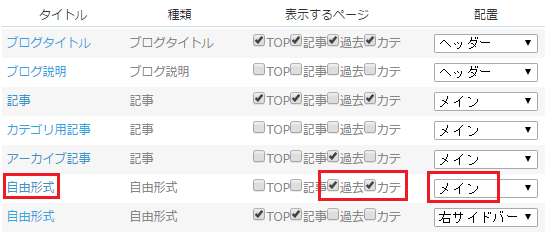※ エキスパートモードで表示しています

アーカイブとカテゴリページはこのように簡単に設定できますが、タグページは HTML 設定を直接変更して、以下の『タグページでのみ表示される内容』の記述方法で設定する必要があります

<% if:page_name eq 'archive' -%>
アーカイブページでのみ表示される内容
<% /if %>

<% if:page_name eq 'category' -%>
カテゴリページでのみ表示される内容
<% /if %>

<% if:page_name eq 'tag' -%>
タグページでのみ表示される内容
<% /if %>

この記述は、以下の場所で使用します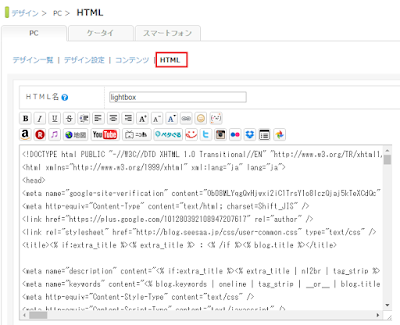```北海道（札幌）北海道（函館）北海道（旭川）北海道（帯広）北海道（釧路）北海道（北見）北海道（室蘭）青森岩手秋田山形宮城福島栃木群馬茨城東京神奈川埼玉千葉山梨新潟長野石川富山福井静岡愛知岐阜三重和歌山奈良滋賀京都大阪兵庫徳島愛媛高知香川岡山広島鳥取島根山口福岡佐賀熊本長崎大分宮崎鹿児島沖縄